Poisson formula

The same as Poisson integral.

A formula giving an integral representation for the solution of the Cauchy problem for the wave equation in: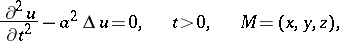This solution has the form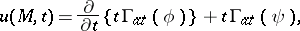(1)

whereis the mean value of the functionon the sphere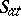in the-space of radius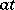and centre at the point, andis the area element on the unit sphere. In the case of the inhomogeneous wave equation a third term is added to formula (1) (see ).

From formula (1), by the method of descent (cf. Descent, method of) formulas are obtained for solving the Cauchy problem in two- (Poisson's formula) and one- (d'Alembert formula) dimensional space (see ). See also Kirchhoff formula.

Sometimes the phrase "Poisson formula" is used for the integral representation of the solution to the Cauchy problem for the heat equation in the space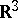: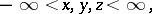This solution has the form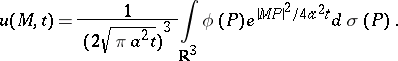(2)

Formula (2) immediately generalizes to an-dimensional space,.